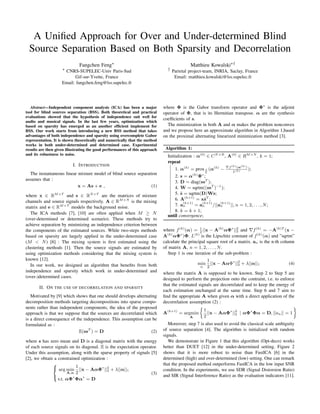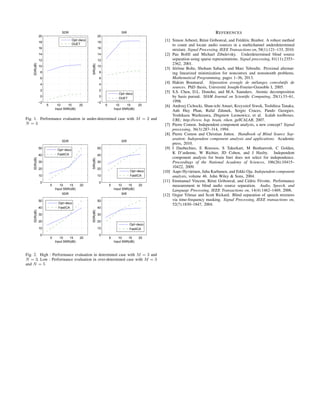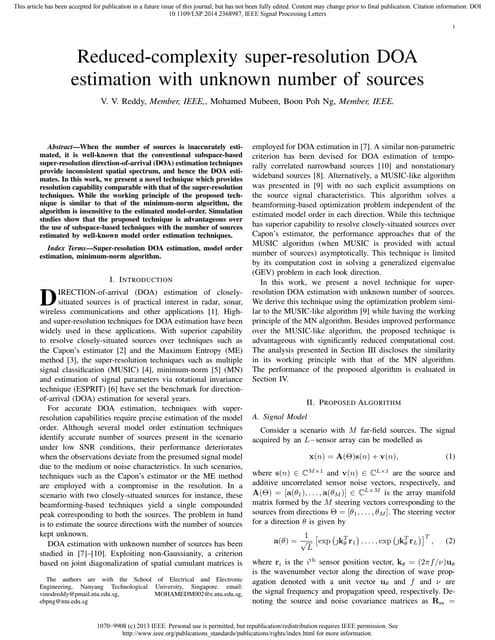AnúncioPróximos SlideShares2015_Reduced-Complexity Super-Resolution DOA Estimation with Unknown Number o...
Carregando em ... 3
1 de 2
Anúncio

### FK_SPARS15

1. A Uniﬁed Approach for Over and Under-determined Blind Source Separation Based on Both Sparsity and Decorrelation Fangchen Feng∗ ∗ CNRS-SUPELEC-Univ Paris-Sud Gif-sur-Yvette, France Email: fangchen.feng@lss.supelec.fr Matthieu Kowalski∗† † Parietal project-team, INRIA, Saclay, France Email: matthieu.kowalski@lss.supelec.fr Abstract—Independent component analysis (ICA) has been a major tool for blind sources separation (BSS). Both theoretical and practical evaluations showed that the hypothesis of independence suit well for audio and musical signals. In the last few years, optimization which based on sparsity has emerged as an another efﬁcient implement for BSS. Our work starts from introducing a new BSS method that takes advantages of both independence and sparsity using overcomplete Gabor representation. It is shown theoretically and numerically that the method works in both under-determined and determined case. Experimental results are then given illustrating the good performances of this approach and its robustness to noise. I. INTRODUCTION The instantaneous linear mixture model of blind source separation assumes that : x = As + e , (1) where x ∈ RM×T and s ∈ RN×T are the matrices of mixture channels and source signals respectively. A ∈ RM×N is the mixing matrix and e ∈ RM×T models the background noise. The ICA methods ,  are often applied when M ≥ N (over-determined or determined scenario). These methods try to achieve separation by minimizing an independence criterion between the components of the estimated sources. While two-steps methods based on sparsity are largely applied in the under-determined case (M < N)  : The mixing system is ﬁrst estimated using the clustering methods . Then the source signals are estimated by using optimization methods considering that the mixing system is known . In our work, we designed an algorithm that beneﬁts from both independence and sparsity which work in under-determined and (over-)determined cases. II. ON THE USE OF DECORRELATION AND SPARSITY Motivated by  which shows that one should develops alternating decomposition methods targeting decompositions into sparse compo- nents rather than independent components, the idea of the proposed approach is that we suppose that the sources are decorrelated which is a direct consequence of the independence. This assumption can be formulated as : E(ssT ) = D (2) where s has zero mean and D is a diagonal matrix with the energy of each source signals on its diagonal. E is the expectation operator. Under this assumption, along with the sparse property of signals  , we obtain a constrained optimization :    arg min A,α 1 2 ||x − AαΦ∗ ||2 2 + λ||α||1 s.t. αΦ∗ Φα∗ = D (3) where Φ is the Gabor transform operator and Φ∗ is the adjoint operator of Φ, that is its Hermitian transpose. α are the synthesis coefﬁcients of s. The minimization in both A and α makes the problem nonconvex and we propose here an approximate algorithm in Algorithm 1,based on the proximal alternating linearized minimization method . Algorithm 1: Initialization : α(0) ∈ CN×B , A(0) ∈ RM×N , k = 1; repeat 1. α(k) = prox λ L (α(k) − f(k) (α(k) ) L(k) ); 2. s = α(k) Φ∗ ; 3. D = diag(ssT ); 4. W = sqrtm((ssT )−1 ); 5. ˜s = sqrtm(D)Ws; 6. A(k+1) = x˜sT ; 7. a (k+1) n = a (k+1) n /||a (k+1) n ||, n = 1, 2, . . . , N; 8. k = k + 1; until convergence; where f(k) (α) = 1 2 ||x − A(k) αΦ∗ ||2 2 and f(k) = −A(k)T (x − A(k) αΦ∗ )Φ. L(k) is the Lipschitz constant of f(k) (α) and ”sqrtm” calculate the principal square root of a matrix. an is the n-th column of matrix A, n = 1, 2, . . . , N. Step 1 is one iteration of the sub-problem : min α 1 2 ||x − AαΦ∗ ||2 2 + λ||α||1 (4) where the matrix A is supposed to be known. Step 2 to Step 5 are designed to perform the projection onto the contraint, i.e. to enforce that the estimated signals are decorrelated and to keep the energy of each estimation unchanged at the same time. Step 6 and 7 aim to ﬁnd the appropriate A when given α with a direct application of the decorrelation assumption (2) : A(k+1) = argmin A 1 2 ||x − AαΦ∗ ||2 2 | αΦ∗ Φα = D, ||an|| = 1 Moreover, step 7 is also used to avoid the classical scale ambiguity of source separation . The algorithm is initialized with random signals. We demonstrate in Figure 1 that this algorithm (Opt-deco) works better than DUET  in the under-determined setting. Figure 2 shows that it is more robust to noise than FastICA  in the determined (high) and over-determined (low) setting. One can remark that the proposed method outperforms FastICA in the low input SNR condition. In the experiments, we use SDR (Signal Distorsion Ratio) and SIR (Signal Interference Ratio) as the evaluation indicators .
2. 5 10 15 20 −2 0 2 4 6 8 10 12 14 16 18 20 SDR Input SNR(dB) SDR(dB) 5 10 15 20 −2 0 2 4 6 8 10 12 14 16 18 20 Input SNR(dB) SIR(dB) SIR Opt−deco DUET Opt−deco DUET Fig. 1. Performance evaluation in under-determined case with M = 2 and N = 3 5 10 15 20 0 10 20 30 40 50 Input SNR(dB) SDR(dB) SDR 5 10 15 20 0 10 20 30 40 50 Input SNR(dB) SIR(dB) SIR 5 10 15 20 0 10 20 30 40 50 Input SNR(dB) SDR(dB) SDR 5 10 15 20 0 10 20 30 40 50 Input SNR(dB) SDR(dB) SIR Opt−deco FastICA Opt−deco FastICA Opt−deco FastICA Opt−deco FastICA Fig. 2. High : Performance evaluation in determined case with M = 3 and N = 3. Low : Performance evaluation in over-determined case with M = 3 and N = 5 REFERENCES  Simon Arberet, R´emi Gribonval, and Fr´ed´eric Bimbot. A robust method to count and locate audio sources in a multichannel underdetermined mixture. Signal Processing, IEEE Transactions on, 58(1):121–133, 2010.  Pau Boﬁll and Michael Zibulevsky. Underdetermined blind source separation using sparse representations. Signal processing, 81(11):2353– 2362, 2001.  J´erˆome Bolte, Shoham Sabach, and Marc Teboulle. Proximal alternat- ing linearized minimization for nonconvex and nonsmooth problems. Mathematical Programming, pages 1–36, 2013.  Hakim Boumaraf. S´eparation aveugle de m´elanges convolutifs de sources. PhD thesis, Universit´e Joseph-Fourier-Grenoble I, 2005.  S.S. Chen, D.L. Donoho, and M.A. Saunders. Atomic decomposition by basis pursuit. SIAM Journal on Scientiﬁc Computing, 20(1):33–61, 1998.  Andrzej Cichocki, Shun-ichi Amari, Krzysztof Siwek, Toshihisa Tanaka, Anh Huy Phan, Rafal Zdunek, Sergio Cruces, Pando Georgiev, Yoshikazu Washizawa, Zbigniew Leonowicz, et al. Icalab toolboxes. URL: http://www. bsp. brain. riken. jp/ICALAB, 2007.  Pierre Comon. Independent component analysis, a new concept? Signal processing, 36(3):287–314, 1994.  Pierre Comon and Christian Jutten. Handbook of Blind Source Sep- aration: Independent component analysis and applications. Academic press, 2010.  I Daubechies, E Roussos, S Takerkart, M Benharrosh, C Golden, K D’ardenne, W Richter, JD Cohen, and J Haxby. Independent component analysis for brain fmri does not select for independence. Proceedings of the National Academy of Sciences, 106(26):10415– 10422, 2009.  Aapo Hyv¨arinen, Juha Karhunen, and Erkki Oja. Independent component analysis, volume 46. John Wiley & Sons, 2004.  Emmanuel Vincent, R´emi Gribonval, and C´edric F´evotte. Performance measurement in blind audio source separation. Audio, Speech, and Language Processing, IEEE Transactions on, 14(4):1462–1469, 2006.  Ozgur Yilmaz and Scott Rickard. Blind separation of speech mixtures via time-frequency masking. Signal Processing, IEEE transactions on, 52(7):1830–1847, 2004.
Anúncio